# How to Add Months to a Date in Excel

In this article, we will learn how to add months and years to date. To add any number of months to a given date we will use EDATE.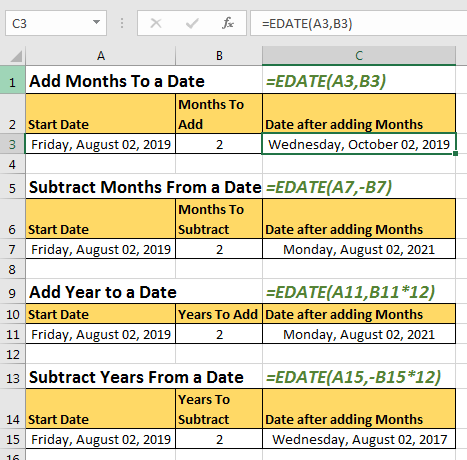#### Generic Formula To Add Months to a Date

=EDATE(start_date,num_of_months)

EDATE function in excel returns a serial number of a future date after adding number of months to a given date.
Start_date: the date to which you want to add months.
Num_of_months: The number of months you want to add to start date.

So without fussing into words lets see an example.

#### How to Add Months To a Start Date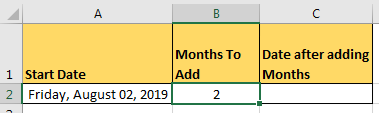For this example, I have this data. In cell A2, there is a start date, in B2 number of months to add and in C2 we will write the formula for adding months to this date.

Write this formula in C2

=EDATE(A2,B2)

This will return the serial number of date which is not much readable for a normal user.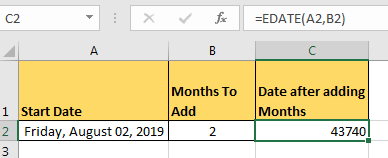To make it readable, change the text format of cell C2 to date. You can copy the format from cell A2 and paste it on C2. Whichever way you like, you do it yourself.
Finally, you’ll have this readable future date.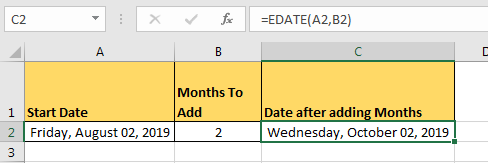#### How to subtract months from a date

To subtract months just add a minus sign before the number of months,

=EDATE(A2,-B2)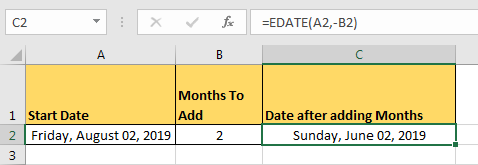#### How to Add Years to a Date

As we all know, each year has 12 months in it. So that’s it. In EDATE function, at the place of num_of_months write the number of years and multiply it by 12.
For example, if I want to add 2 years to Friday, August 02, 2019 I will write this formula to add years in excel.

=EDATE(A2,B2*12)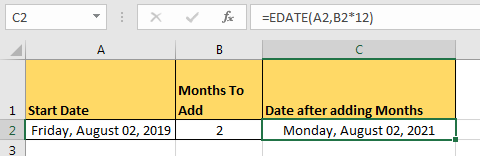The same goes for subtracting years from a date.
So yeah guys, we can add or remove months and years from a date in excel using the excel EDATE function. If you have any doubts, let me know in the comments section below.

Related Articles:

Popular Articles:

50 Excel Shortcuts to Increase Your Productivity

How to use the VLOOKUP Function in Excel

How to use the COUNTIF function in Excel

How to use the SUMIF Function in Excel

Terms and Conditions of use

The applications/code on this site are distributed as is and without warranties or liability. In no event shall the owner of the copyrights, or the authors of the applications/code be liable for any loss of profit, any problems or any damage resulting from the use or evaluation of the applications/code.